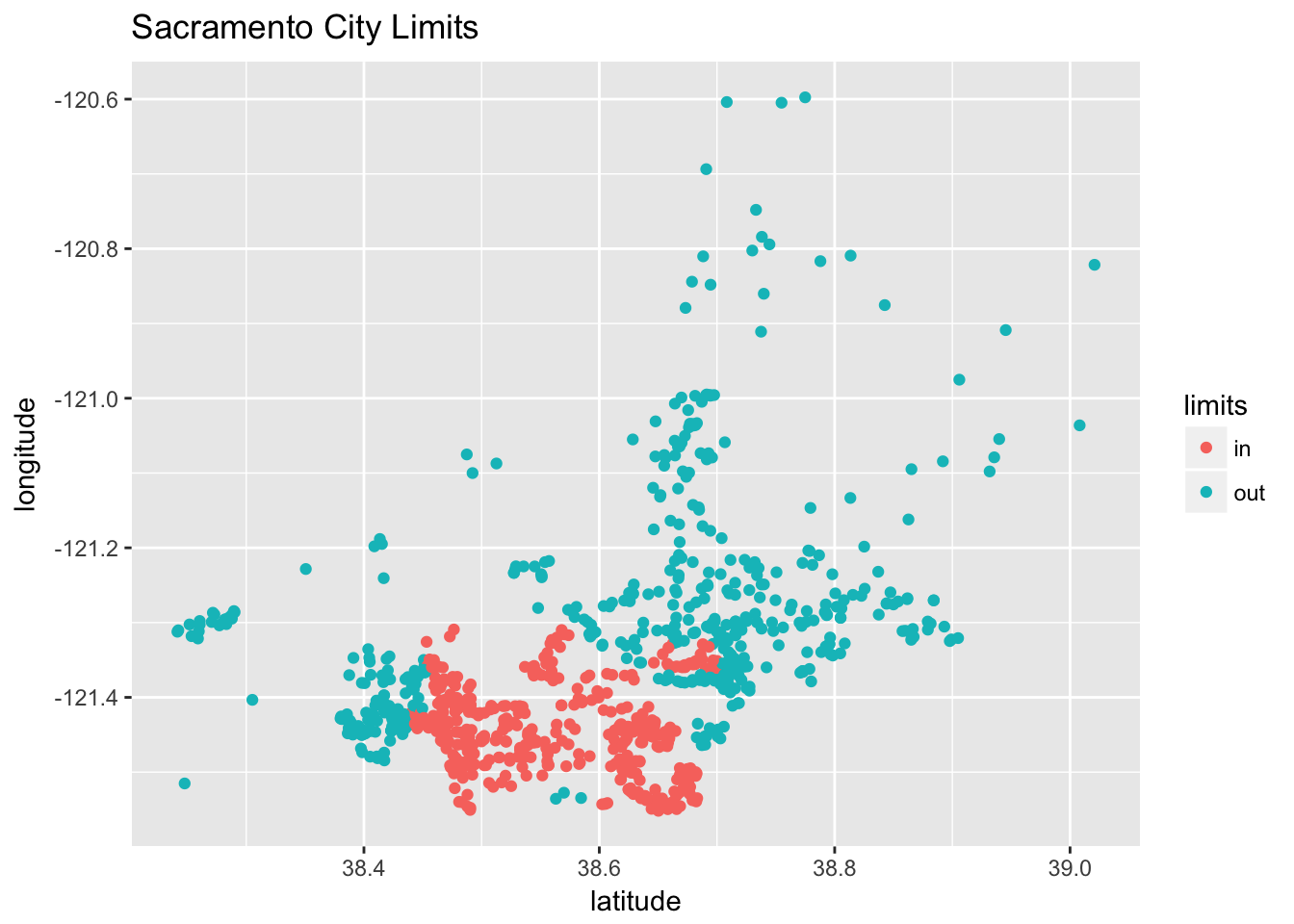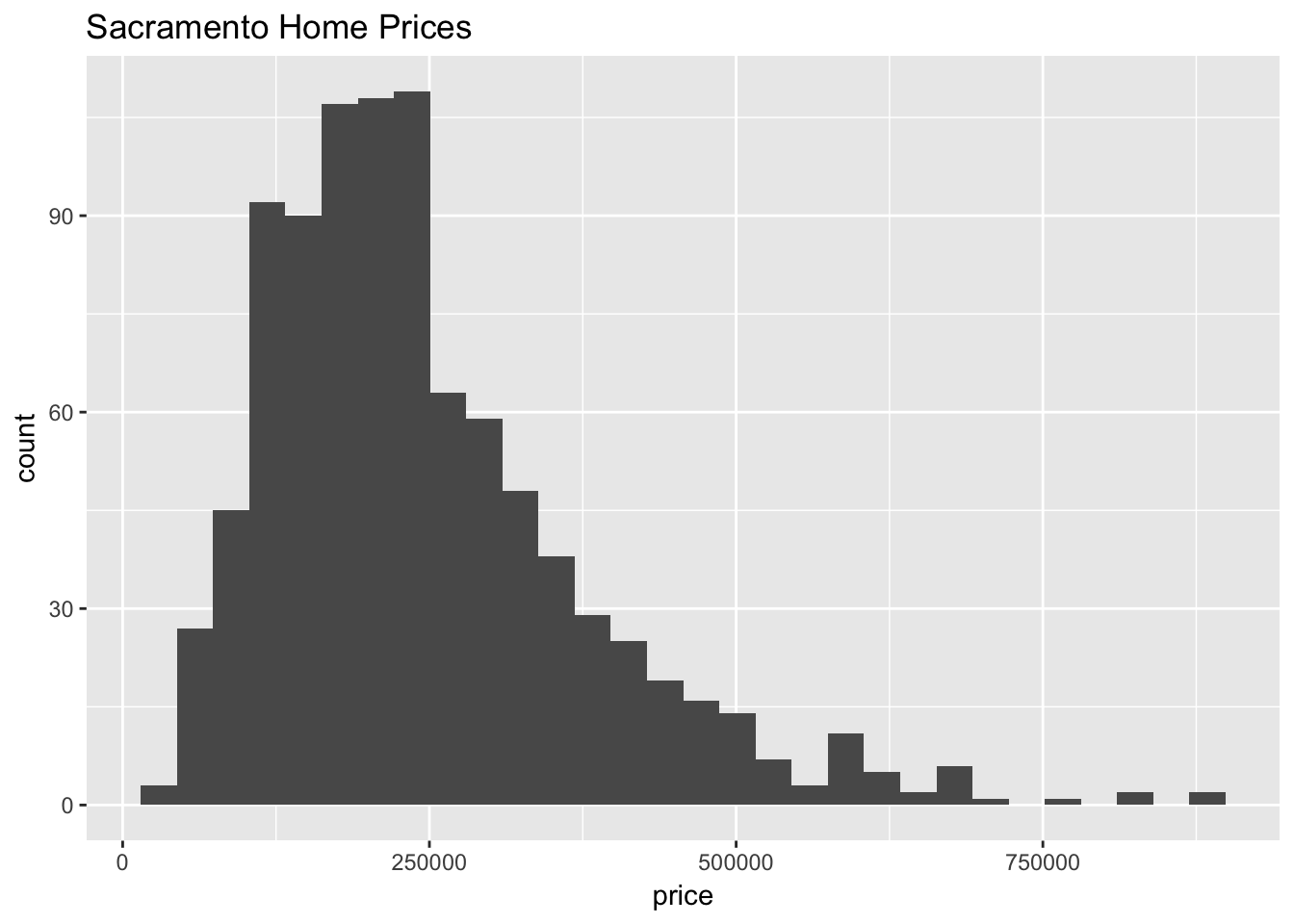For this homework we will again use the `Sacramento` data from the `caret` package. You should read the documentation for this data. The goal of our modeling will be to predict home prices.

You may only use the following packages:

``````library(caret)
library(randomForest)
library(tidyverse)
library(knitr)
library(kableExtra)``````

Before modeling, we will perform some data preparation.

Instead of using the `city` or `zip` variables that exist in the dataset, we will simply create a variable indicating whether or not a house is technically within the city limits Sacramento. (We do this because they would both be factor variables with a large number of factors. This is a choice that is made due to laziness, not because it is justified. Think about what issues these variables might cause.)

``````data(Sacramento)
sac_data = Sacramento
sac_data\$limits = factor(ifelse(sac_data\$city == "SACRAMENTO", "in", "out"))
sac_data = subset(sac_data, select = -c(city, zip))``````

A plot of longitude versus latitude gives us a sense of where the city limits are.

``````qplot(y = longitude, x = latitude, data = sac_data,
col = limits, main = "Sacramento City Limits ")``````You should consider performing some additional exploratory data analysis, but we provide a histogram of the home prices.

``qplot(x = price, data = sac_data, main = "Sacramento Home Prices")``After these modifications, we test-train split the data.

``````set.seed(42)
sac_trn_idx  = sample(nrow(sac_data), size = trunc(0.80 * nrow(sac_data)))
sac_trn_data = sac_data[sac_trn_idx, ]
sac_tst_data = sac_data[-sac_trn_idx, ]``````

The training data should be used for all model fitting. Do not modify the data for any exercise in this assignment.

## Exercise 1 (\(k\)-Nearest Neighbors Preprocessing)

For this exercise, we will create \(k\)-nearest neighbors models in an attempt to be able to predict `price`. Do not modify `sac_trn_data`. Do not use the `baths` variable. Use the `knnreg` function from the `caret` package.

Consider three different preprocessing setups:

• Setup 1
• Numeric variables not scaled.
• Factor variables remain factors. `knnreg()` will use one-hot encoding.
• Setup 2
• Numeric variables are scaled to have mean 0 and standard deviation 1.
• Factor variables remain factors. `knnreg()` will use one-hot encoding.
• Setup 3
• Numeric variables are scaled to have mean 0 and standard deviation 1.
• Factor variables coerced to numeric. (We could then scale them, but for simplicity we will not.)

For each setup, train models using values of `k` from `1` to `100`. For each, calculate test RMSE. (In total you will calculate 300 test RMSEs, 100 for each setup.) Summarize these results in a single plot which plots the test RMSE as a function of `k`. (The plot will have three “curves,” one for each setup.) Your plot should be reasonably visually appealing, well-labeled, and include a legend.

Solution:

``````# helper function for RMSE
calc_rmse = function(actual, predicted) {
sqrt(mean((actual - predicted) ^ 2))
}``````
``````# define values of k to tune over
k = 1:100``````
``````# define model setups
sac_knn_form_1 = as.formula(price ~ beds + sqft + type + latitude + longitude +
limits)
sac_knn_form_2 = as.formula(price ~ scale(beds) + scale(sqft) + type +
scale(latitude) + scale(longitude) + limits)
sac_knn_form_3 = as.formula(price ~ scale(beds) + scale(sqft) + as.numeric(type) +
scale(latitude) + scale(longitude) + as.numeric(limits))``````
``````# fit models for each k within each setup
sac_knn_mod_1 = lapply(k, function(x) {knnreg(sac_knn_form_1, data = sac_trn_data, k = x)})
sac_knn_mod_2 = lapply(k, function(x) {knnreg(sac_knn_form_2, data = sac_trn_data, k = x)})
sac_knn_mod_3 = lapply(k, function(x) {knnreg(sac_knn_form_3, data = sac_trn_data, k = x)})``````
``````# get all predictions
sac_knn_pred_1 = lapply(sac_knn_mod_1, predict, newdata = sac_tst_data)
sac_knn_pred_2 = lapply(sac_knn_mod_2, predict, newdata = sac_tst_data)
sac_knn_pred_3 = lapply(sac_knn_mod_3, predict, newdata = sac_tst_data)``````
``````# calculate test RMSE for each k for each setup
sac_knn_rmse_1 = sapply(sac_knn_pred_1, calc_rmse, actual = sac_tst_data\$price)
sac_knn_rmse_2 = sapply(sac_knn_pred_2, calc_rmse, actual = sac_tst_data\$price)
sac_knn_rmse_3 = sapply(sac_knn_pred_3, calc_rmse, actual = sac_tst_data\$price)``````